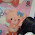Saturday, October 16, 2010

2010 SPM Exams Tips - Physics

Paper 2

Section A:

Question 1: Form 4 (Chapter 1)

Measuring Instrument - Vernier calliper/micrometer screw gauge (reading, accuracy, zero error)

Question 2: Form 5 (Chapter 2)

Choose type of fuse

Electric circuit for safety

Question 3: Form 4 (Chapter 4)

Specific latent heat of fusion and Specific latent heat of vaporisation

Steaming of food

Question 4: Form 4 (Chapter 3)

Bernoulli principle (Aerofoil)

Question 5: Form 5 (Chapter 3)

Electromagnet (electric bell)

Question 6: Form 5 (Chapter 1)

Refraction of water

Question 7: Form 5 (Chapter 5)

Equation for alpha, beta and gamma decay (Graph of nucleon number against proton number)

Calculation for the nuclear energy

Question 8: Form 4 (Chapter 2)

Principle of conservation of energy (definition and calculation)

Choose spring (Most elastic)

Section B: (Modify and explain)

Question 9-Essay: Form 4 (Chapter 5)

Compare size of image formed by different object distance

Application of convex mirror. (widen the viewing angle)

Modify projector @ microscope @ telescope)

Question 10-Essay: Form 5 (Chapter 3)

Electromagnetic induction (Lenz Law and Faraday law, calculation, graph, AC generator, factors)

Design DC adaptor from AC supply (transformer and rectifier)

Section C: (Choose suitability)

Question 11-Essay: Form 4 (Chapter 5)

Archimedes’s principle (definition, calculation, float concept calculation)

Choose material for hot air balloon

Question 12-Essay: Form 5 (Chapter 4)

Semiconductor and transistor as automatic switch

Choose material for semiconductor

Paper 3

Section A:

Question 1: Form 4 (Chapter 5)

Snell Law/Refractive index (sin i against sin r) (Reading on protractor)

Question 2: Form 5 (Chapter 2)

Internal resistance (E = V + Ir)

Section B: (Practical)

1. Form 4 (Chapter 2)

Mass and Acceleration experiment (Ticker timer)

2. Form 4 (Chapter 4)

Gas Law experiment (Boyle’s Law, Charles’s Law and Pressure Law)

Specific Heat Capacity experiment (mass water increases, change in temperature decreases)

3. Form 5 (Chapter 1)

Interference of Light or Sound experiment (a increases, x decreases)

4. Form 5 (Chapter 2)

Factors that affect Resistance (length, cross-sectional area, temperature and type of material)

Ohm’s Law experiment (V = IR)

List of Experiemts:

Form 4 – Chapter 2

(1) To investigate the relationship between inertia and mass

(2) To investigate the relationship between force and acceleration

(3) To investigate the relationship between mass and acceleration

Form 4 – Chapter 3

(4) To investigate the relationship between depth and pressure of liquid

(5) To investigate the relationship between immersion destance in water and weight

Form 4 – Chapter 4

(6) To investigate the relationship between mass of water and change in temperature

(7) To investigate the relationship between the pressure and the volume (Boyle’s Law)

(8) To determine the relationship between the pressure and the temperature (Pressure Law)

(9) To determine the relationship between the volume and the temperature (Charles’ Law)

Form 5 – Chapter 1

(10) To investigate the relationship between the distance between two coherent source and distance bwteen two consecutive constructive inteference (Light and sound)

Form 5 – Chapter 2

(11) To investigate the relationship between current and the potential difference for a wire

(12) To investigate the factors affecting resistance (length, thickness of wire and temperature

1.2.3.4.